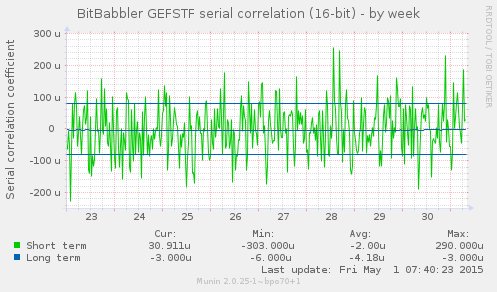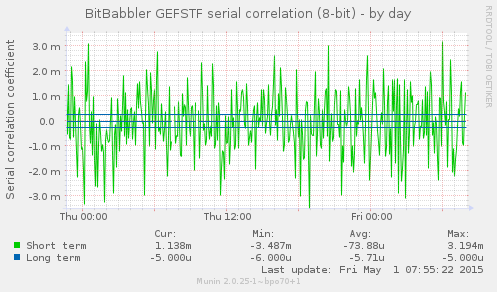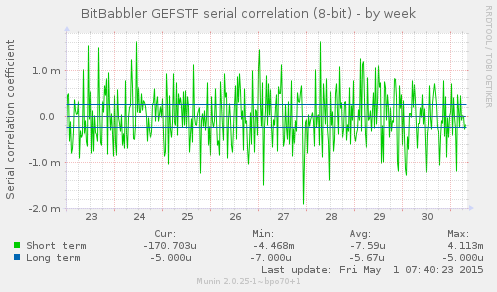# ENT test results

### Chi-squared distribution

This first set of graphs show the results of Pearson's Chi-squared test for the distribution of values in short and long sequences of 8 and 16-bit samples. The short term results test a block of the most recently generated samples, while the long term result is computed over all of the samples that have been generated since the monitoring process began.

For the short block tests, which are independent, the 5% and 0.1% thresholds are directly relevant, with results expected to be outside of those on approximately that proportion of trials. A correctly functioning device is expected to produce results which are randomly distributed through the whole of the probable range. A value smaller than the lower threshold indicates a result which is more uniform than would normally be expected, while a value larger than the higher thresholds is the result of a greater than usual deviation from the expected distribution. Both extremes are expected to occur in some small percentage of trials.

A sustained rate of results outside of these bounds for the short term test would indicate a systemic failure. The results of the long term test are a bit trickier to interpret, since it is continually accumulating upon the same set of data so each new result is not independent of what came before it. As such it may be expected to take fairly long duration excursions out to the extreme limits of probability before eventually returning to a more expected range. It likewise is expected to cover the entire probable range, but as the number of samples that it is based on increases, its probable rate of change will slow. A RNG that is genuinely bad in a way that is not sufficiently uniform (but by a small enough margin that it does not immediately trigger other test failures) is likely to put the long term result into a state of perpetual increase that does not slow or turn around as it reaches the outer limits of (im)probability. The long term result becomes increasingly sensitive to small abnormalities as the number of samples grow.### Estimated entropy

This next set of graphs show the calculated Shannon and min entropy for a short term sequence of the most recent samples, and over the long term for all samples generated since the monitoring process began.

The Shannon entropy is based on the number of times that each possible sequence of 8 or 16 bits occurred. The min-entropy is a more conservative estimate that is based only on the number of times that the most frequent sample value was seen. The 'entropy' value shown by the original command line `ent` tool is the Shannon entropy. Calculation of min-entropy is something that we have added, but plot on the same graph.

The results shown here are after a long enough period of running that the long term results for Shannon entropy have reached their asymptote, but when recording first begins that will start at some lower value and gradually increase to approach it. The long term min-entropy is still slowly increasing at this point.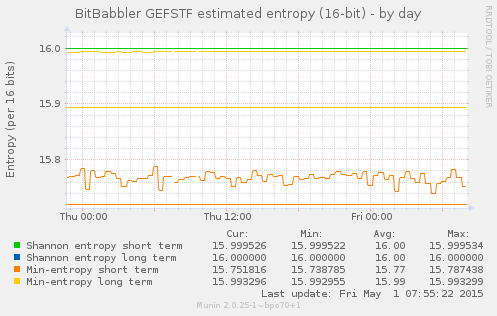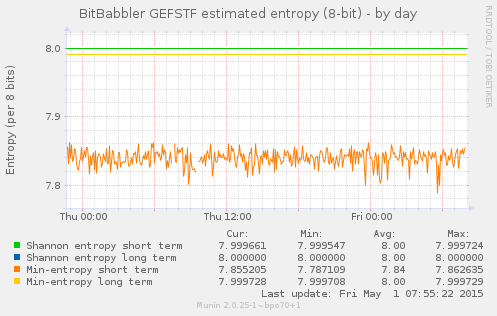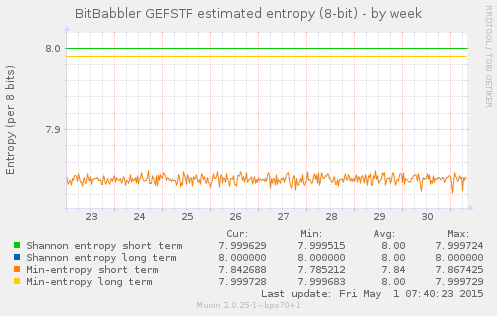### Arithmetic mean

These graphs show the simple arithmetic mean of 8 and 16-bit samples over short and long term sequences. The short term result is a test of the most recently generated samples. The long term result is calculated over all samples generated since the monitoring process began. An unbiased sequence would be expected to converge on 127.5 and 32767.5 over the long term, but it may take a very large number of samples to do so, particularly for the 16-bit average. The short term results are all independent tests, so they are expected to always deviate randomly from that, but the average of those values are also expected to approach the median over time as well.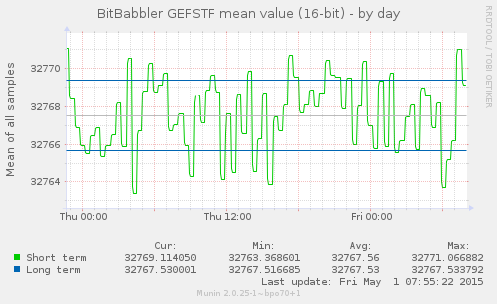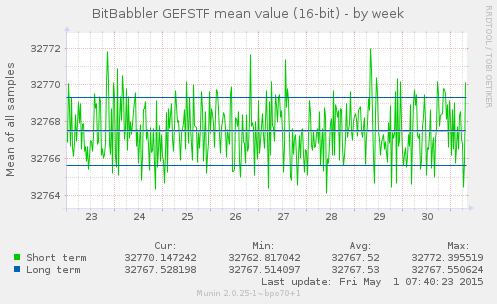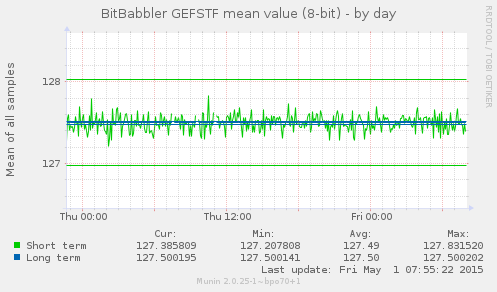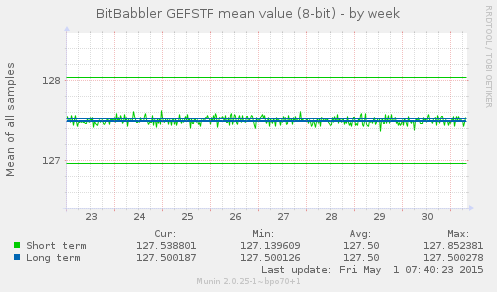### Monte Carlo estimation of π

These graphs show the error in computing the value of π using the 'Monte Carlo Method'. Consecutive sequences of 24 bits are taken as X and Y coordinates inside a square. Since a circle inscribed in that square occupies π/4 of its area, then a uniformly distributed set of random points should fall inside or outside the radius of the circle with a ratio that when multiplied by 4 gives an approximation for π. The short term result is a test of the most recently generated samples. The long term result is computed over all samples generated since the monitoring process began.

The results are graphed as the percentage of error relative to the real value of π. This test is relatively slow to converge on an accurate estimation, but a sustained or persistently diverging inaccuracy in the estimation would indicate a systemic error in the uniformity of the sample values. As with the arithmetic mean, the short term values are expected to be scattered on both sides of zero error but will average very nearly to it – if they are consistently too near to it, that is as much a warning of some problem causing poor randomness as being too far from it is.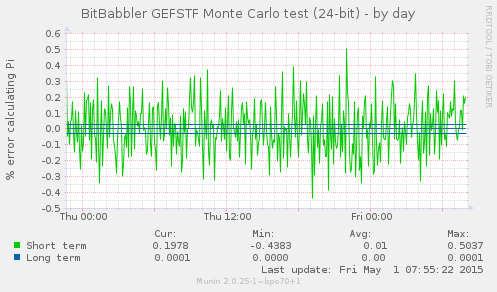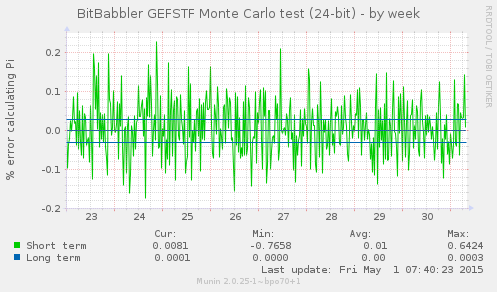### Serial correlation

These graphs show the autocorrelation coefficient for a lag of 1 over the sequence of samples. This gives a measure of the extent to which each sample is related to the previous one. A perfectly predictable stream will converge on a result of 1.0, and a perfectly unpredictable one will converge on a result of 0.

The short term result is a test of the most recently generated samples. The long term result is computed over all samples generated since the monitoring process began. A sustained divergence away from 0 or values close to ±1 indicate a problem that ought to be investigated.